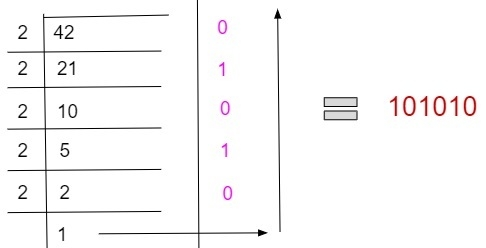# Program for Decimal to Binary Conversion in C++

Given with a decimal number as an input, the task is to convert the given decimal number into a binary number.

Decimal number in computers is represented with base 10 and binary number is represented with base 2 as it has only two binary digits 0 and 1 whereas decimal numbers can be any numeric digit starting from 0 – 9.

To convert a decimal number into a binary number follow the given steps −

• Firstly divide the given number with the base value of conversion number e.g. dividing 42 by 2 because we need to convert 42 into a binary numbers which have base 2 and then obtain a quotient and store it. If the remainder is 0 store the bit as 0 else 1.
• Divide the obtained quotient with the base value of binary number which is 2 and keep storing the bits.
• Keep doing right shift to the stored bits
• Repeat the step until the remainder left indivisible

Given below is the pictorial representation of converting a decimal number into a binary number.## Example

Input-: 42
Divide the 42 with base 2 : 42 / 2 = 0 (remainder) 21(quotient)
Divide quotient with base: 21 / 2 = 1(remainder) 10(quotient)
Divide quotient with base: 10 / 2 = 0(remainder) 5(quotient)
Divide quotient with base: 5 / 2 = 1(remainder) 2(quotient)
Divide quotient with base: 2 / 2 = 0(remainder) 1(quotient)
Now reverse the bits to obtain final value.
Output-: 101010

## Algorithm

Start
Step 1-> declare function to convert decimal to binary
int convert(int num)
Loop For int i = 31 i >= 0 i—
Set int k = num >> i
If (k & 1)
Print "1"
End
Else
Print "0"
End
End
Step 2-> In main()
Declare and set int num = 42
Call convert(num)
Stop

## Example

Live Demo

#include <iostream>
using namespace std;
//convert decimal to binary
int convert(int num) {
for (int i = 31; i >= 0; i--) {
int k = num >> i;
if (k & 1)
cout << "1";
else
cout << "0";
}
}
int main() {
int num = 42;
convert(num);
}

## Output

IF WE RUN THE ABOVE CODE IT WILL GENERATE FOLLOWING OUTPUT

00000000000000000000000000101010